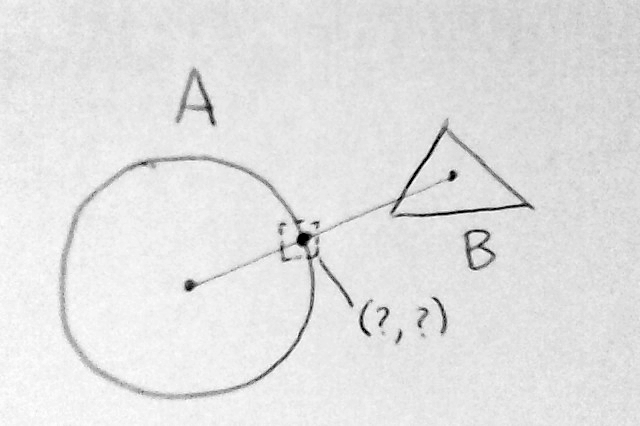# Calculate the intersection between a line and a sprite edge

How could I get the 2D coordinates of the intersection between a sprite “A” and a line that’s a projection from the center of another sprite “B” to the center of A?

BTW, Could it be done without adding colliders or any extra component to the involved sprites?EDIT: I drew a circle and a triangle by chance, shapes of the sprites maybe different.

If we are talking about a circle and triangle kind of “vector shapes”, it’s not terribly complicated to do this, but if the shapes can change dynamically (you can assign different PNG’s to the objects in code) and you expect pixel perfect collisions/coordinates that match the contents of the PNG’s, there’s no magic bullet to solve this.

You somehow need to know the coordinates of the edges of your shapes (a sequence of line segments that define the “hull” of the object ). After that the problem is solvable.

This should be a working C# implementation for checking if 2 line segments intersect

``````    /// <summary>
/// Checks if a line between p0 to p1 intersects  a line between p2 and p3
///
/// returns the point of intersection
/// </summary>
public static Vector2? lineSegmentsIntersectPos(Vector2 p0, Vector2 p1, Vector2 p2, Vector2 p3)
{
float s1_x, s1_y, s2_x, s2_y;

s1_x = p1.x - p0.x;
s1_y = p1.y - p0.y;
s2_x = p3.x - p2.x;
s2_y = p3.y - p2.y;

float s, t;
s = (-s1_y * (p0.x - p2.x) + s1_x * (p0.y - p2.y)) / (-s2_x * s1_y + s1_x * s2_y);
t = (s2_x * (p0.y - p2.y) - s2_y * (p0.x - p2.x)) / (-s2_x * s1_y + s1_x * s2_y);

if (s >= 0 && s <= 1 && t >= 0 && t <= 1)
{
// 			 Collision detected
return new Vector2(p0.x + (t * s1_x), p0.y + (t * s1_y));
}

return null; // No collision

}
``````

For line to circle collisions a different formula is more optimal.

I didn’t find a way to do it without colliders, so I guess it is impossible.

With colliders is quite easy. You can set the collider in the editor so it fits the shape of the sprite, and then you just have to cast a ray and get the point of the hit.

Something like this:

``````Vector2 intersect;

RaycastHit2D[] hits = Physics2D.RaycastAll (A.transform.position, B.transform.position);
foreach (RaycastHit2D hit in hits) {
if (hit.collider = theColliderIamLookingFor) {
intersect = hit.point;
}
}
``````